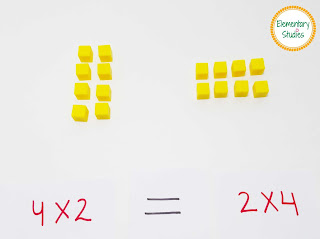## Elementary Studies

### Properties of Multiplication

In continuation of my posts on the Multiplication topic, today I will be sharing my work on Properties of Multiplication. As per common core standards a 3rd grader is expected to know about these multiplication properties which includes Associative property, Commutative property, Distributive property, Identity property, and Zero property.

This week when I was teaching this topic, I had my kiddo first use the blocks to visualize and see these multiplication properties in action. I am a big fan of these place value blocks and it always comes handy. I bought mine from Lakeshore, but these are now available at Amazon for less 😊. You can get these at Amazon: Place Value Blocks

Later, I gave her the worksheets (HERE) to reinforce her understanding of Properties of Multiplication.

Associative Property of multiplication states that you can change the grouping of the factors the product will be the same.
Example: (5 x 2) x 3 = 30
5 x (2 x 3) = 30

Commutative Property of multiplication states that you can multiply the factors in any order, the product stays the same.
Example: 4 x 2 = 2 x 4Commutative Property of Multiplication

Distributive Property of multiplication states that when one factor of multiplication is rewritten as sum of two numbers, the product does not change.
Example:  6 x 5 =    6 x ( 3 + 2)
=   (6 x 3) + ( 6 x 2)
=    18 + 12
=    30Distributive Property of Multiplication

Identity Property of multiplication states that if a number is multiplied by 1, the product is the number itself.
Example: 5 x 1 = 5

Zero Property of multiplication states that the product of any number and zero is zero.
Example: 7 x 0 = 0

Learning these properties can be quite overwhelming for kids and it will be a good idea to have them create a foldable which they can glue in their interactive notebooks or take home for review.

### Properties of Multiplication Worksheets

I have created 7 worksheets to go with this topic. You can find these HERE.

Thanks,
Shimps